# Price elasticity of demand

(Redirected from Price-elasticity of demand)

## Definition

### Backdrop

The price elasticity of demand is defined with the following backdrop:

• The specific good, service, or commodity.
• A certain set of economic actors who are the potential buyers of that commodity.
• An economic backdrop that includes all the determinants of demand other than the unit price of that commodity.

### Point price elasticity

The price elasticity at a price$p$ is defined in any of the following four equivalent ways: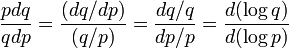$\! \frac{pdq}{q dp} = \frac{(dq/dp)}{(q/p)} = \frac{dq/q}{dp/p} = \frac{d(\log q)}{d(\log p)}$

If we want to emphasize the fact that the quantity demanded is, in fact, a function not just of the unit price but of a number of other factors, then we can use partial derivative symbols: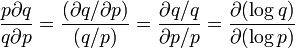$\! \frac{p\partial q}{q \partial p} = \frac{(\partial q/\partial p)}{(q/p)} = \frac{\partial q/q}{\partial p/p} = \frac{\partial(\log q)}{\partial(\log p)}$

In other words, it is the ratio of the rate of change of quantity demand with respect to price, to the ratio of quantity to price. Note that the price elasticity of demand is a number; it has no units and is independent of the units used to measure the price or quantity demanded.

### Interval price elasticity

The price elasticity between a price$p_1$ (with corresponding quantity demanded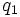$q_1$) and a price$p_2$ (with corresponding quantity demanded$q_2$).

Conceptually, the interval price elasticity can be thought of as follows:$\frac{\mbox{Fractional change in quantity}}{\mbox{Fractional change in price}}$

Or equivalently, as: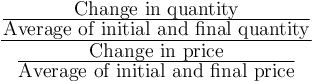$\frac{\frac{\mbox{Change in quantity}}{\mbox{Average of initial and final quantity}}}{\frac{\mbox{Change in price}}{\mbox{Average of initial and final price}}}$

This simplifies to: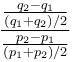$\frac{\frac{q_2 - q_1}{(q_1 + q_2)/2}}{\frac{p_2 - p_1}{(p_1 + p_2)/2}}$

The above can be simplified into any of these equivalent forms:$\frac{(q_2 - q_1)/(q_2 + q_1)}{(p_2 - p_1)/(p_2 + p_1)} = \frac{(p_1 + p_2)(q_2 - q_1)}{(q_1 + q_2)(p_2 - p_1)} = \frac{(q_2 - q_1)/(p_2 - p_1)}{(q_2 + q_1)/(p_2 + p_1)}$

### Interpretation in terms of demand curve

The price elasticity of demand is the reciprocal of the slope of the logarithmic demand curve -- the variant of the demand curve that plots the logarithm of price on the vertical axis and the logarithm of quantity demanded on the vertical axis.

### Distinction from cross-price elasticity of demand

Price elasticity of demand is sometimes referred to as own-price elasticity of demand to distinguish it from cross-price elasticity of demand which measures the sensitivity of the demand for one commodity to changes in the price of another commodity, and is used to judge the extent to which the commodities are complementary goods or substitute goods.

## Properties

### Sign

The law of demand says that the price elasticity of demand is zero or negative at all prices. Thus, a positive price elasticity indicates a violation (or apparent violation) of the law of demand.

Price elasticities of demand are often reported as positive numbers. Unless it is clearly indicated that the law of demand is violated in the particular case, these positive numbers are to be interpreted as the magnitudes of the corresponding price elasticities and the actual value of the price elasticity is negative.

### Interpretation of various values of price elasticity

Note that all price elasticities here are zero or negative, and to avoid unnecessary negative signs, we state results in terms of the magnitude of price elasticity.

Constraint on magnitude of price elasticity at a particular price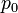$p_0$ Interpretation in terms of demand curve Interpretation in terms of total money spent on the good (see also total revenue test) Term used Type of good
Magnitude equals$0$ The demand curve is vertical (or has a vertical tangent) at the price level of$p_0$ The total money spent is directly proportional to the price The demand is completely inelastic Typically, a necessity good or a basic necessity
Magnitude between$0$ and$1$ The demand curve is downward-sloping; although not vertical, it is steeper than the price elasticity$1$ case The total money spent increases with an increase in price, though the increase is less than proportional to the price increase The demand is inelastic, but not completely A necessity good, though with more flexibility in the decision of how much to consume
Magnitude of$1$ The demand curve is downward-sloping, shape like a rectangular hyperbola The total money spent does not change with changes in price around$p_0$  ?  ?
Magnitude greater than$1$ The demand curve is downward-sloping, though less steep than the price elasticity$1$ case The total money spent decreases with an increase in price because the drop in quantity demanded more than cancels the effect of the greater unit price The demand is highly elastic Typically, a luxury good, consumption of which is optional and is hence more price-sensitive.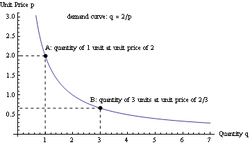For this demand curve, the quantity demanded is inversely proportional to the price, hence the price elasticity of demand is -1, or its magnitude is 1

Note that necessity goods and luxury goods are defined in terms of their income elasticity of demand; however, the general principle remains that higher magnitudes of price elasticity of demand correlate with higher magnitudes of income elasticity of demand.

### Substitution effect and impact on price elasticity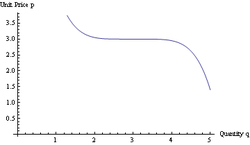This demand curve has a very high price elasticity around a price level of 3 units; the price elasticity becomes less at higher as well as lower prices.

The presence of close substitutes has the following implication: at prices close to the market prices of close substitutes, the price elasticity of demand is very high. At prices much higher than the market prices of close substitutes, demand is uniformly low so the price elasticity of demand is relatively low. At prices much lower than the market prices of close substitutes, demand is high, and the price elasticity of demand is again relatively low.

## Effect of price elasticity on other things

### Effect on sensitivity of price to supply shifts

The higher the price elasticity of demand, the lower the sensitivity of market price to shifts of the supply curve.

Thus, for necessity goods (such as staple foods and, in the case of petroleum-dependent societies, petroleum products), which have a low price elasticity of demand, the market price is much more sensitive to shifts in supply. In particular, a small supply shock can cause a significant increase in price. The main explanation for this massive shift is that, because of the relative inelasticity of demand to price, the price needs to be increased significantly to induce people to cut down on demand in order to compensate for the reduction in supply.

In contrast, for non-essential goods, which generally have a high price elasticity of demand, supply shifts do not generally lead to significant price changes, because small (fractional) changes in price are sufficient to induce buyers to cut back on demand.